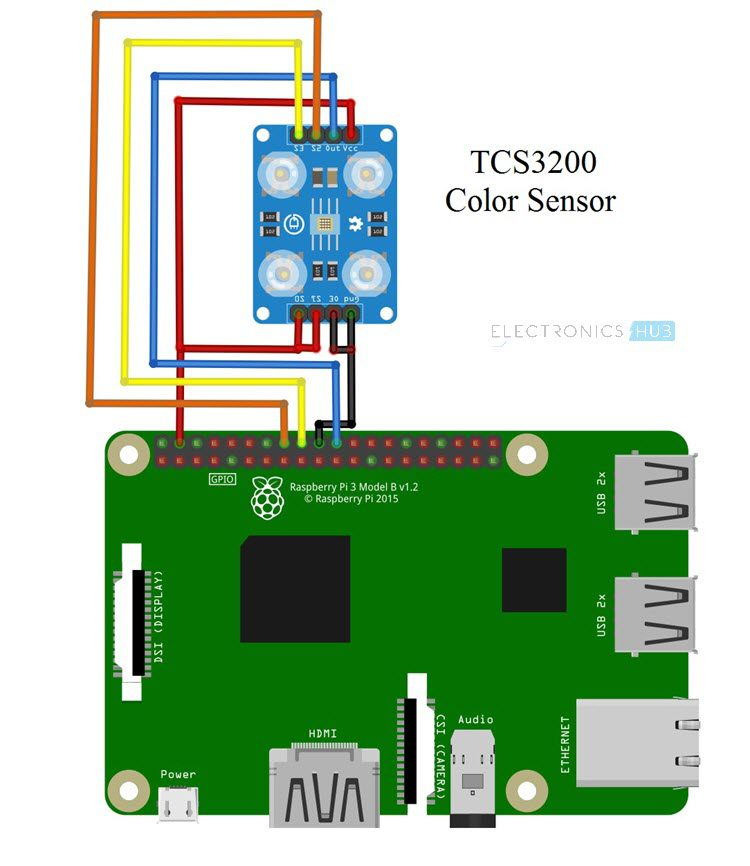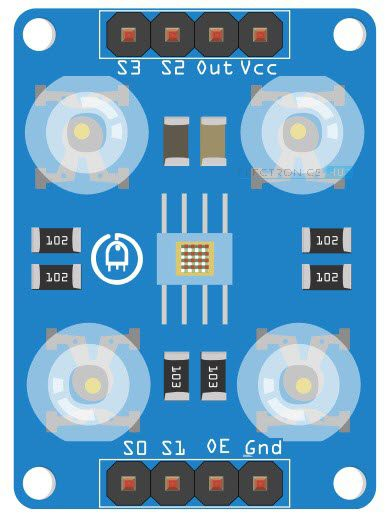# Raspberry Pi Color Sensor Tutorial | Color Detector using TCS3200Continuing further in our Raspberry Pi exploration, we will do a project on Raspberry Pi based Color Detection. In this project, we will learn about TCS3200 Color Sensor and how the Raspberry Pi Color Sensor (TCS3200, to be specific) interface can be implemented for Color Detection.

### Overview

Color Sensor detects or senses color. It is as simple as that. But how the Color Sensor actually detects the color is what makes things interesting.

There are many applications like object detection, product sorting, object tracking etc. All these and many other applications have Color Detection as a part of their system.

In color detection process, the Color Sensor is the main components or hardware. There are several types of Color Sensors that are used for different applications.

In the Raspberry Pi Color Sensor Interface Tutorial, I’ll be using the TCS3200 Color Sensor from TAOS (Texas Advanced Optoelectronic Solutions).

### A Brief Note on TCS3200 Color Sensor

I have already made a project on TCS3200 Color Sensor. It is based on Arduino and the project is called ARDUINO BASED COLOR DETECTION.

In that project, I have spoken about basics of color sensors, how an LDR can be used as a Color Sensor and also a brief introduction to TCS3200 Color Sensor.So, I strongly recommend you to go through that project (at least the Introduction to Color Sensor part) before continuing with Raspberry Pi Color Sensor Interface.

TCS3200 is one of the easily available color sensor that students and hobbyists can work on. It is basically a light to frequency converter i.e. based on color and intensity of the light falling on it, the frequency of its output signal varies.

### Pin Diagram of TCS3200

The following image shows the Pin Diagram of TCS3200. It is available in 8-pin SOIC Package.### Pin Description of TCS3200

• S0 and S1: S0 and S1 are Output Signal Frequency Scaling inputs. Using these pins, you can scale the output frequency to one of the three preset values. Table corresponding to S0 and S1 is given in the later sections.
• OE: It is the Output Enable Pins. It is an active LOW pin.
• GND: Power Supply Ground Pin.
• VDD: Power Supply Pin (usually, +5V).
• OUT: Output Pin, which produces a square ware of 50% Duty cycle and the frequency of the square wave is proportional to the intensity of the light.
• S2 and S3: S2 and S3 are the Photo Diode Selection Pins.

NOTE: The following table shows the different combinations of S0 and S1 inputs and corresponding output frequency scaling.

 S0 S1 Output Frequency Scaling (f0) Typical full-scale Frequency L L Power Down ———- L H 2% 10 – 12 KHz H L 20% 100 – 120 KHz H H 100% 500 – 600 KHz

The table below shows the combination of inputs S2 and S3 and the corresponding Photo Diode selected.

 S3 S4 Photodiode Type L L Red L H Blue H L Clear (no filter) H H Green

### Circuit Diagram of Raspberry Pi Color Sensor Interface

The following Fritzing based image shows the connections diagram of the Raspberry Pi Color Sensor (TCS3200) interface.If the Pin Out of the TCS3200 Color Sensor in the above circuit diagram is not clear, you can use the following image as a reference.### Components Required

• Raspberry Pi
• TCS3200 Color Sensor
• Connecting Wires
• Power Supply
• Computer

### Circuit Design

As mentioned earlier, TCS3200 Color Sensor has 8-pins. Connect Pins 1 and 2 i.e. S0 and S1 to +5V. Then connect theand GND to gnd of the Raspberry Pi.

Connect the VDD pin of the TCS3200 Color Sensor to +5V of Raspberry Pi. Pin 6 i.e. OUT pin of the Sensor is connected to Physical Pin 22 i.e. GPIO25 of Raspberry Pi.

Finally, connect the S2 and S3 of the color sensor to physical pins 16 and 18 i.e. GPIO23 and GPIO24 of the Raspberry Pi.

NOTE: Use a 5V Adapter to power the Raspberry Pi instead of connecting it to a computer.

### Code

#### Code for RAW RGB Values

I’ll provide two codes for this project. Using the first code, you can display the RAW values of the RGB Gamut.

 import RPi.GPIO as GPIO import time s2 = 23 s3 = 24 signal = 25 NUM_CYCLES = 10 def setup(): GPIO.setmode(GPIO.BCM) GPIO.setup(signal,GPIO.IN, pull_up_down=GPIO.PUD_UP) GPIO.setup(s2,GPIO.OUT) GPIO.setup(s3,GPIO.OUT) print(“\n“) def loop(): temp = 1 while(1): GPIO.output(s2,GPIO.LOW) GPIO.output(s3,GPIO.LOW) time.sleep(0.3) start = time.time() for impulse_count in range(NUM_CYCLES): GPIO.wait_for_edge(signal, GPIO.FALLING) duration = time.time() – start #seconds to run for loop red = NUM_CYCLES / duration #in Hz print(“red value – “,red) GPIO.output(s2,GPIO.LOW) GPIO.output(s3,GPIO.HIGH) time.sleep(0.3) start = time.time() for impulse_count in range(NUM_CYCLES): GPIO.wait_for_edge(signal, GPIO.FALLING) duration = time.time() – start blue = NUM_CYCLES / duration print(“blue value – “,blue) GPIO.output(s2,GPIO.HIGH) GPIO.output(s3,GPIO.HIGH) time.sleep(0.3) start = time.time() for impulse_count in range(NUM_CYCLES): GPIO.wait_for_edge(signal, GPIO.FALLING) duration = time.time() – start green = NUM_CYCLES / duration print(“green value – “,green) time.sleep(2) def endprogram(): GPIO.cleanup() if __name__==‘__main__’: setup() try: loop() except KeyboardInterrupt: endprogram()

#### Code for Color Detection using Raspberry Pi and TCS3200 Color Sensor

Using the second code, you can implement a Color Detection Project. The code is calibrated to only three primary colors: Red, Green and Blue. But you can expand to a wide range of colors based on the results from the first code.

 import RPi.GPIO as GPIO import time s2 = 23 s3 = 24 signal = 25 NUM_CYCLES = 10 def setup(): GPIO.setmode(GPIO.BCM) GPIO.setup(signal,GPIO.IN, pull_up_down=GPIO.PUD_UP) GPIO.setup(s2,GPIO.OUT) GPIO.setup(s3,GPIO.OUT) print(“\n“) def loop(): temp = 1 while(1): GPIO.output(s2,GPIO.LOW) GPIO.output(s3,GPIO.LOW) time.sleep(0.3) start = time.time() for impulse_count in range(NUM_CYCLES): GPIO.wait_for_edge(signal, GPIO.FALLING) duration = time.time() – start red = NUM_CYCLES / duration GPIO.output(s2,GPIO.LOW) GPIO.output(s3,GPIO.HIGH) time.sleep(0.3) start = time.time() for impulse_count in range(NUM_CYCLES): GPIO.wait_for_edge(signal, GPIO.FALLING) duration = time.time() – start blue = NUM_CYCLES / duration GPIO.output(s2,GPIO.HIGH) GPIO.output(s3,GPIO.HIGH) time.sleep(0.3) start = time.time() for impulse_count in range(NUM_CYCLES): GPIO.wait_for_edge(signal, GPIO.FALLING) duration = time.time() – start green = NUM_CYCLES / duration if green<7000 and blue<7000 and red>12000: print(“red”) temp=1 elif red<12000 and blue<12000 and green>12000: print(“green”) temp=1 elif green<7000 and red<7000 and blue>12000: print(“blue”) temp=1 elif red>10000 and green>10000 and blue>10000 and temp==1: print(“place the object…..”) temp=0 def endprogram(): GPIO.cleanup() if __name__==‘__main__’: setup() try: loop() except KeyboardInterrupt: endprogram()

### Working

The aim of this simple project is to understand the Raspberry Pi Color Sensor Interface and how can we make a Color Detection application using Raspberry Pi and TCS3200 Color Sensor.

Now, coming to the working, since both the S0 and S1 inputs of the TCS3200 Color Sensor are connected to +5V, the output frequency is scaled to 100% i.e. the output frequency will be in the range of 500 KHz to 600 KHz.

As S2 and S3 pins of the TCS3200 Color Sensor are used to select the Photo Diode, they are set in three different combination one after the other to get the RAW data of the Red, Blue and Green values.

Keeping these values as reference, the color detection program is written, where the Raspberry Pi correctly displays the name of the color placed in front of the sensor.

NOTE:

• The reference values in the second Python Script are dependent on the surrounding lighting. So, they may not work perfectly for you.
• In order to make a Color Detection Application, implement the first Python Script and note down values. Based on these values, you can develop your own Python Script for the Color Detector Application.

### Applications

As already mentioned in the beginning, Color Sensors like TCS3200 can be implemented in a variety of projects and applications like:

• RGB LED Backlight Control
• Object Color Verification
• Product Sorting
• Industrial Automation
• Commercial Printing
• Health and Fitness

## Similar Articles & Blogs

Explore similar articles on various electronics and electrical topics –